# Precalculus : Matrices and Vectors

## Example Questions

### Example Question #7 : Multiplication Of Matrices

Compute: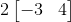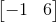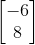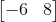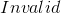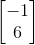Explanation:

A scalar that multiplies a one by two matrix will result in a one by two matrix.

Multiply the scalar value with each value in the matrix.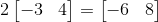### Example Question #1 : Matrices

Evaluate: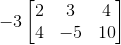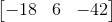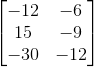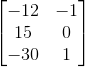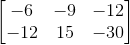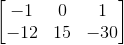Explanation:

This problem involves a scalar multiplication with a matrix. Simply distribute the negative three and multiply this value with every number in the 2 by 3 matrix. The rows and columns will not change.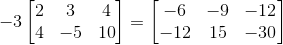### Example Question #1 : Matrices

Simplify: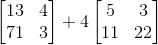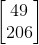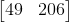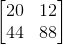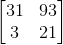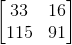Explanation:

Scalar multiplication and addition of matrices are both very easy. Just like regular scalar values, you do multiplication first: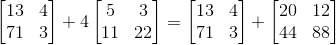The addition of matrices is very easy. You merely need to add them directly together, correlating the spaces directly.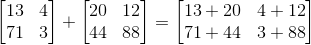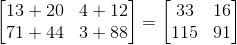### Example Question #1 : Matrices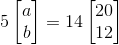What is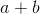?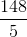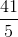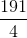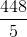Explanation:

You can begin by treating this equation just like it was: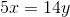That is, you can divide both sides by: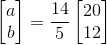Now, for scalar multiplication of matrices, you merely need to multiply the scalar by each component: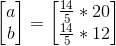Then, simplify: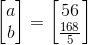Therefore,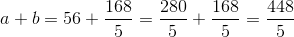### Example Question #41 : Matrices And Vectors

Find 3A given: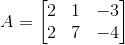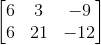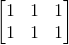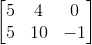Not possibleExplanation:

To multiply a scalar and a matrix, simly multiply each number in the matrix by the scalar. Thus,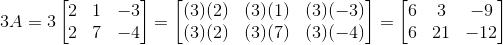### Example Question #1 : How To Find Scalar Interactions With A Matrix

If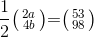, what is?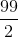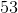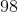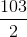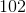Explanation:

Begin by distributing the fraction through the matrix on the left side of the equation. This will simplify the contents, given that they are factors of: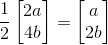Now, this means that your equation looks like: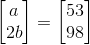This simply means: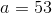and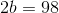or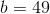Therefore,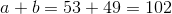### Example Question #1 : Find The Product Of Two Matrices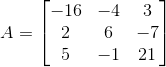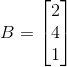Find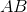No SolutionExplanation:

The dimensions of A and B are as follows: A= 3x3, B= 3x1

When we mulitply two matrices, we need to keep in mind their dimensions (in this case 3x3 and 3x1).

The two inner numbers need to be the same. Otherwise, we cannot multiply them. The product's dimensions will be the two outer numbers: 3x1.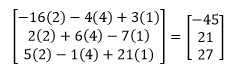### Example Question #2 : Find The Product Of Two Matrices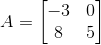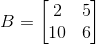FindNo SolutionExplanation:

The dimensions of both A and B are 2x2. Therefore, the matrix that results from their product will have the same dimensions.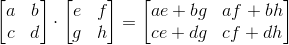Thus plugging in our values for this particular problem we get the following: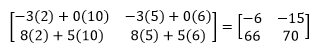### Example Question #3 : Find The Product Of Two Matrices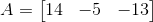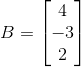Find.No SolutionExplanation:

The dimensions of A and B are as follows: A=1x3, B= 3x1.

Because the two inner numbers are the same, we can find the product.

The two outer numbers will tell us the dimensions of the product: 1x1.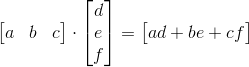Therefore, plugging in our values for this problem we get the following: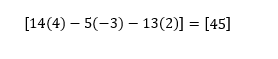### Example Question #1 : Find The Product Of Two Matrices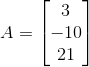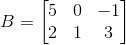Find.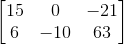No Solution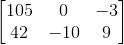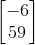No Solution

Explanation:

The dimensions of A and B are as follows: A= 3x1, B= 2x3

In order to be able to multiply matrices, the inner numbers need to be the same. In this case, they are 1 and 2. As such, we cannot find their product.

The answer is No Solution

### All Precalculus Resources